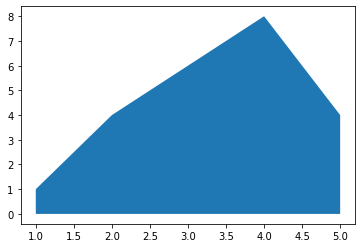There are 2 main functions to draw a basic area chart using matplotlib: `fill_between()` and `stackplot()` functions. I advise the `fill_between()` function which allows easier customisation. The `stackplot()` function also works, but it is more adapted for stacked area charts. The inputs are 2 numerical variables(x and y values) for both functions.

``````# libraries
import numpy as np
import matplotlib.pyplot as plt

# Create data
x=range(1,6)
y=[1,4,6,8,4]

# Area plot
plt.fill_between(x, y)

# Show the graph
plt.show()

# Note that we could also use the stackplot function
# but fill_between is more convenient for future customization.
#plt.stackplot(x,y)
#plt.show()``````## Contact & Edit

👋 This document is a work by Yan Holtz. You can contribute on github, send me a feedback on twitter or subscribe to the newsletter to know when new examples are published! 🔥